# Radioactive decay facts for kids

Kids Encyclopedia Facts

Radioactive decay happens to some chemical elements. Most chemical elements are stable. Stable elements are made up of atoms that stay the same. Even in a chemical reaction, the atoms themselves do not change ever.

In the 19th century, Henri Becquerel discovered that some chemical elements have atoms that change over time. In 1898, Marie and Pierre Curie called this phenomenon radioactive decay. Becquerel and the Curies were awarded the Nobel Prize in Physics for this discovery, in 1903.

## Example

Most carbon atoms have six protons and six neutrons in their nucleus. This carbon is called carbon-12s (six protons + six neutrons = 12). Its atomic weight is 12. If a carbon atom has two more neutrons it is carbon-14. Carbon-14 acts chemically like other carbon, because the six protons and six electrons are what govern its chemical properties. In fact, carbon-14 exists in all living things; all plants and animals contain carbon-14. However, carbon-14 is radioactive. It decays by beta decay to become nitrogen-14. Carbon-14, in the small amounts found about us in nature, is harmless. In archeology, this kind of carbon is used to determine the age of wood and other formerly living things. The method is called radiocarbon dating.

## Different kinds of decay

Ernest Rutherford found that there are different ways in which these particles penetrate matter. He found two different kinds, which he called alpha decay and beta decay. Paul Villard discovered a third kind in 1900. Rutherford called it gamma decay, in 1903.

The change from radioactive carbon-14 to stable nitrogen-14 is a radioactive decay. It happens when the atom emits an alpha particle. An alpha particle is a pulse of energy as an electron or positron leaves the nucleus.

Other kinds of decay were discovered later. The types of decay are different from each other because different types of decay produce different kinds of particles. The starting radioactive nucleus is called the parent nucleus and the nucleus that it changes into is called the daughter nucleus. The high-energy particles produced by radioactive materials are called radiation.

These various kinds of decay can happen sequentially in a "decay chain". One kind of nucleus decays to another kind, which decays again to another and so on until it becomes a stable isotope and the chain comes to an end.

## Speed of decay

The speed at which this change happens, is different for each element. Radioactive decay is governed by chance: The time it takes, on average for half the atoms of a substance to change is named the half-life. The rate is given by an exponential function. As an example, iodine (131I) has a half-life of about 8 days. That of plutonium ranges between 4 hours (243Pu) and 80 million years (244Pu)

## Nuclear transformations and energy

Radioactive decay changes an atom from one that has higher energy inside its nucleus into one with lower energy. The change of energy of the nucleus is given to the particles that are created. The energy released by radioactive decay may either be carried away by a gamma ray electromagnetic radiation (a type of light), a beta particle or an alpha particle. In all those cases, the change of energy of the nucleus is carried away. And in all those cases, the total number of positive and negative charges of the atom's protons and electrons sum to zero before and after the change.

## Alpha decay

During alpha decay, the atomic nucleus releases an alpha particle. Alpha decay causes the nucleus to lose two protons and two neutrons. Alpha decay causes the atom to change into another element, because the atom loses two protons (and two electrons). For example, if Americium were to go through alpha decay it would change into Neptunium because Neptunium is defined by having two protons fewer than Americium. Alpha decay usually happens in the most heavy elements, such as uranium, thorium, plutonium, and radium.

Alpha particles cannot even go through a few centimeters of air. Alpha radiation cannot hurt humans when the alpha radiation source is outside the human body, because human skin does not let the alpha particles go through. Alpha radiation can be very harmful if the source is inside the body, such as when people breathe dust or gas containing materials which decay by emitting alpha particles (radiation).

## Beta decay

There are two kinds of beta decay, beta-plus and beta-minus.

In beta-minus decay, the nucleus gives out a negatively charged electron and a neutron changes into a proton: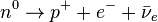$n^0 \rightarrow p^+ + e^- + \bar{\nu}_e$.
where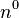$n^0$ is the neutron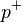$\ p^+$ is the proton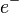$e^-$ is the electron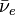$\bar{\nu}_e$ is the anti-neutrino

Beta-minus decay happens in nuclear reactors.

In beta-plus decay, the nucleus releases a positron, which is like an electron but it is positively charged, and a proton changes into a neutron: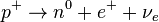$\ p^+ \rightarrow n^0 + e^+ + {\nu}_e$.
where$\ p^+$ is the proton$n^0$ is the neutron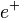$e^+$ is the positron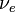${\nu}_e$ is the neutrino

Beta-plus decay happens inside the sun and in some types of particle accelerators.

## Gamma decay

Gamma decay happens when a nucleus produces a high-energy packet of energy called a gamma ray. Gamma rays do not have electrical charge, but they do have angular momentum. Gamma rays are usually emitted from nuclei just after other types of decay. Gamma rays can be used to see through material, to kill bacteria in food, to find some types of disease, and to treat some kinds of cancer. Gamma rays have the highest energy of any electromagnetic wave, and gamma ray bursts from space are the most energetic releases of energy known.

## Images for kidsRadioactive decay Facts for Kids. Kiddle Encyclopedia.